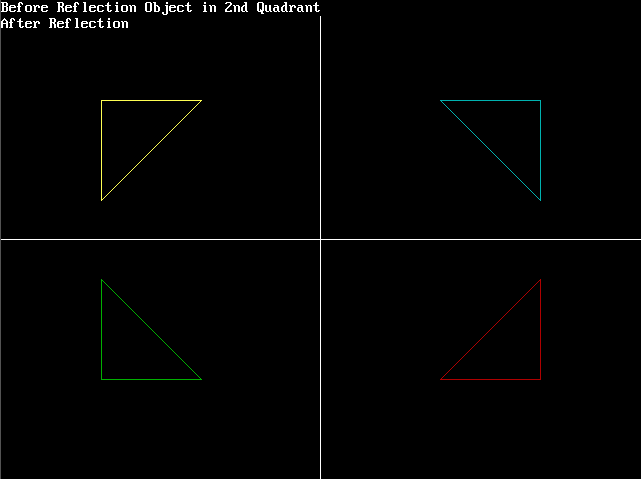# C program to perform reflection of the given 2D image using computer graphics

• Difficulty Level : Medium
• Last Updated : 04 May, 2021

In this article, we will discuss how to perform reflection in C using computer graphics using logic rather than the direct matrix formula of translation followed by rotation and translation.

This technique has currently been applied for the object taken as a triangle, the code can be applied to any object, provided the (Xn, Yn) coordinates are adapted into it.

The base logic of this technique is that in any given reflection, the lateral inversion of the reflected object is at the same distance from any given side as the source object. i.e., if a source object is at a distance of 10 pixels from the left, then the reflected object will be at a distance of 10 pixels from the right and the same is applicable for top and bottom sides.

Example on a real-life basis:

• If a person is standing in front of a mirror, the distance between the right hand of the person and the mirror’s right boundary will be the same as the distance between the left hand of the person’s reflection and the left boundary.
• For the convenience of displaying the above technique via a program let’s draw the source object at the 2nd graph quadrant, but it can be done from any quadrant with minor changes to the code.

Steps of the aforementioned technique:

• Create an object in the 2nd graph quadrant by providing the coordinates.
• For reflection along X-axis:
• Y-axis coordinates will remain the same.
• Obtain laterally inverted X-axis coordinates distance by calculating the distance between the X coordinate of the source object and its nearest surface along the X-axis.
• Draw another object using the laterally inverted X coordinates obtained from the above step while keeping the Y coordinate the same.
• The above steps will generate a mirror image or reflection of the source object.
• In this scenario, the reflected object will be formed at the 1st quadrant.
•  For reflection along Y-axis:
• X-axis coordinates will remain the same.
• Obtain laterally inverted Y-axis coordinates’ distance by calculating the distance between the Y coordinate of the source object and its nearest surface along the Y-axis.
• Draw another object using the laterally inverted Y coordinates obtained from the above step while keeping the X coordinate the same.
• The above steps will generate a mirror image or reflection of the source object.
• In this scenario, the reflected object will be formed at the 3rd quadrant.
• 2c. For reflection along the origin:
• X-axis and Y-axis coordinates will change.
• Obtain laterally inverted Y-axis coordinate distance by calculating the distance between the Y coordinate of the source object and its nearest surface along the Y-axis.
• Obtain laterally inverted X-axis coordinate distance by calculating the distance between the X coordinate of the source object and its nearest surface along the X-axis.
• Draw another object using the laterally inverted X and Y coordinates obtained from the above steps.
• The above steps will generate a mirror image or reflection of the source object.
• This time, the reflection is about origin as both X & Y coordinates have changed, pushing the object inside out.
• In this scenario, the reflected object will be formed at the 4th quadrant.

Approach:

1. Draw a line in graphics to act as a Y-axis, by passing along 4 values as parameters of the line() function as line(getmaxx()/2, 0, getmaxx()/2, getmaxy()).
2. Draw a line in graphics to act as X-axis by passing along 4 values as parameters of the line() function as line(0, getmaxy()/2, getmaxx(), getmaxy()/2).
3. Draw an object using the line() function with parameters from set variables.
4. Perform reflection and draw the object along origin using Step 3 of the technique and color it red to distinguish it from other objects.
5. Perform reflection and draw the object along the X-axis using Step 1 of the technique and color it cyan to distinguish it from other objects.
6. Perform reflection and draw the object along the Y-axis using Step 2 of the technique and color it green to distinguish it from other objects.

Below is the implementation of the above approach:

## C

 `// C program for the above approach`` ` `#include ``#include ``#include `` ` `// Driver Code``void` `main()``{``    ``// Initialize the drivers``    ``int` `gm, gd = DETECT, ax, x1 = 100;``    ``int` `x2 = 100, x3 = 200, y1 = 100;``    ``int` `y2 = 200, y3 = 100;`` ` `    ``// Add in your BGI folder path``    ``// like below initgraph(&gd, &gm,``    ``// "C:\\TURBOC3\\BGI");``    ``initgraph(&gd, &gm, ``""``);``    ``cleardevice();`` ` `    ``// Draw the graph``    ``line(getmaxx() / 2, 0, getmaxx() / 2,``         ``getmaxy());``    ``line(0, getmaxy() / 2, getmaxx(),``         ``getmaxy() / 2);`` ` `    ``// Object initially at 2nd quadrant``    ``printf``(``"Before Reflection Object"``           ``" in 2nd Quadrant"``);`` ` `    ``// Set the color``    ``setcolor(14);``    ``line(x1, y1, x2, y2);``    ``line(x2, y2, x3, y3);``    ``line(x3, y3, x1, y1);``    ``getch();`` ` `    ``// After reflection``    ``printf``(``"\nAfter Reflection"``);`` ` `    ``// Reflection along origin i.e.,``    ``// in 4th quadrant``    ``setcolor(4);``    ``line(getmaxx() - x1, getmaxy() - y1,``         ``getmaxx() - x2, getmaxy() - y2);`` ` `    ``line(getmaxx() - x2, getmaxy() - y2,``         ``getmaxx() - x3, getmaxy() - y3);`` ` `    ``line(getmaxx() - x3, getmaxy() - y3,``         ``getmaxx() - x1, getmaxy() - y1);`` ` `    ``// Reflection along x-axis i.e.,``    ``// in 1st quadrant``    ``setcolor(3);``    ``line(getmaxx() - x1, y1,``         ``getmaxx() - x2, y2);``    ``line(getmaxx() - x2, y2,``         ``getmaxx() - x3, y3);``    ``line(getmaxx() - x3, y3,``         ``getmaxx() - x1, y1);`` ` `    ``// Reflection along y-axis i.e.,``    ``// in 3rd quadrant``    ``setcolor(2);``    ``line(x1, getmaxy() - y1, x2,``         ``getmaxy() - y2);``    ``line(x2, getmaxy() - y2, x3,``         ``getmaxy() - y3);``    ``line(x3, getmaxy() - y3, x1,``         ``getmaxy() - y1);``    ``getch();`` ` `    ``// Close the graphics``    ``closegraph();``}`

Output:My Personal Notes arrow_drop_up